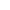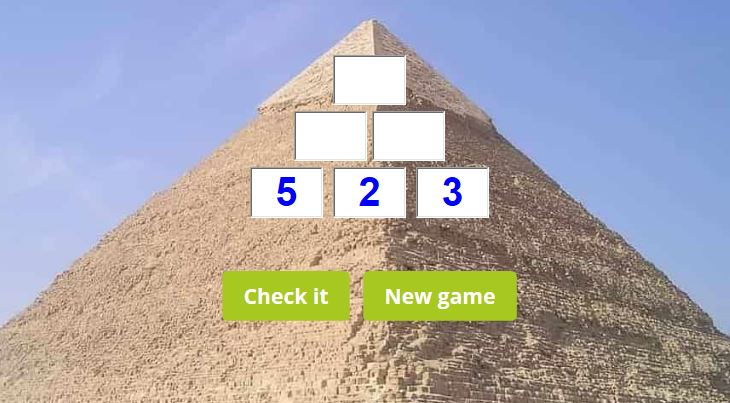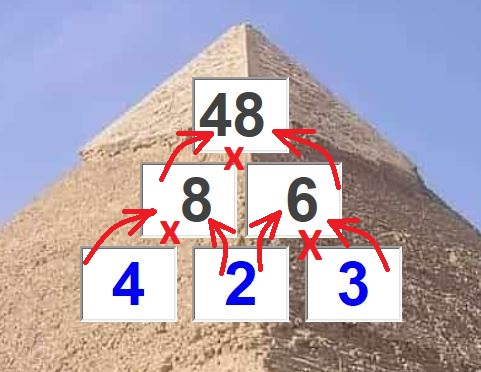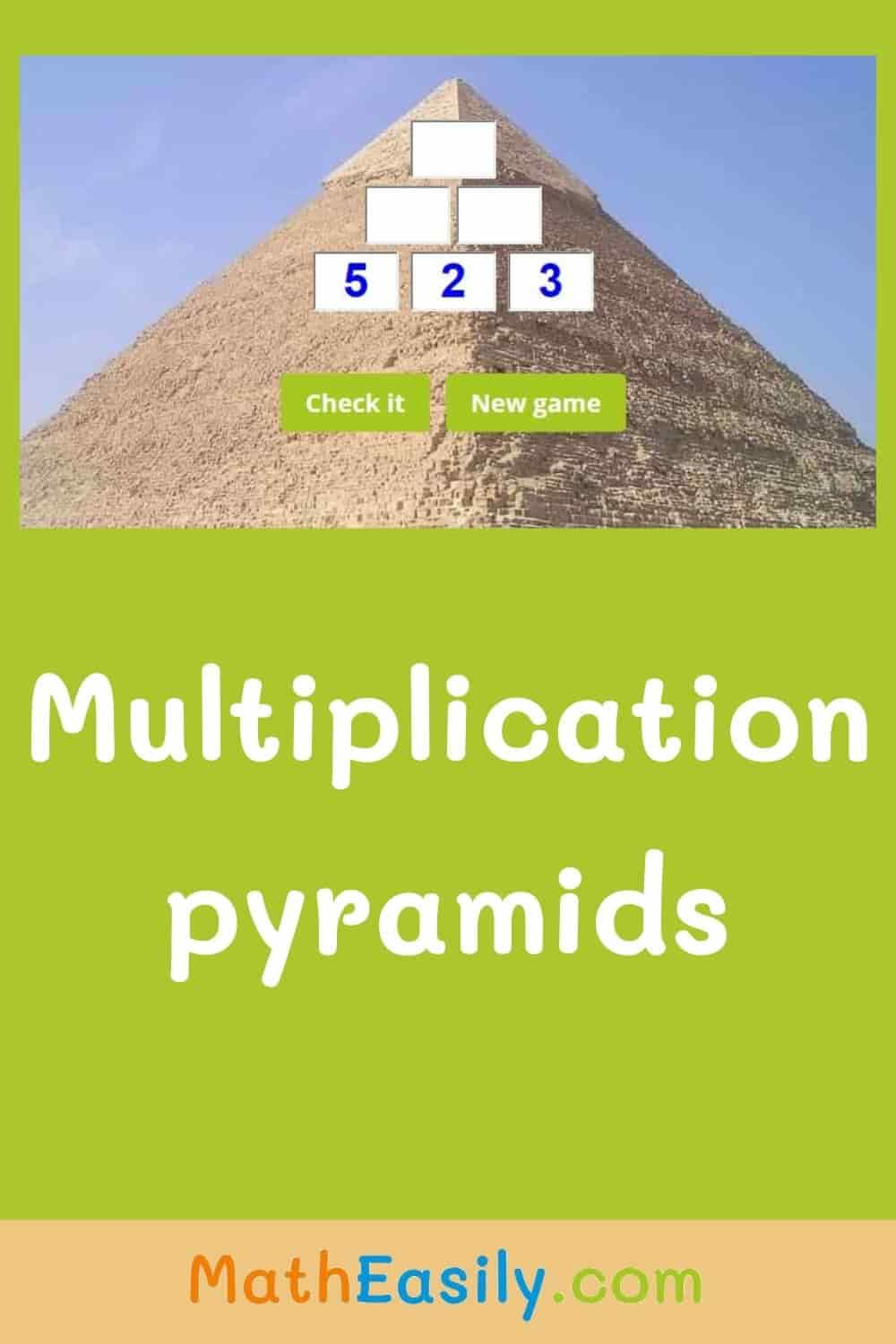﻿ Multiplication Pyramids: Worksheets and Online Games

Page No. 1065

# Multiplication Pyramid Puzzle

Play the multiplication pyramid puzzle. Download multiplication pyramids worksheets. number pyramid multiplication. multiplication pyramid year 5. multiplication pyramid activity. The online multiplication pyramid game.

New game## How to do the multiplication pyramid game?In a multiplication pyramid, numbers next to each other are multiplied to make the number above it.

Insert the result of multiplication to the empty boxes of the multiplication pyramid game. Fill in multiplication pyramids online and learn to multiply up to 500. Choose the maximum product on the top of the pyramid: 100, 300 or 500.Press "Check it" to find out if the result is OK. The correct answers will be green, wrong results will be red. Press the button "New game" and the new multiplication pyramid will be generated.

## Multiplication pyramid worksheet

Generate free multiplication pyramid worksheets in PDF. These multiplication pyramid puzzles consist of two pages: the first page contains 6 multiplication pyramids, the other page includes also the right answers. You can find even more free multiplication worksheets here.

## Why to play multiplication pyramids?

Multiplication pyramid game is a nice way how to learn multiplication. To play this game kids need to have basic knowledge of single digit multiplication.

Do you want to try something more difficult? Then play this two digit by one digit multiplication game.

You can play the multiplication pyramid game in the classroom, at home or anywhere with an internet connection.

### You might also like these number pyramid games:## Multiplication pyramid activity

This multiplication pyramid activity is intended for 3rd grade math students or 4th grade math students. These kids are already familiar with basics of multiplication.

If you want to try another multiplication activity, play these online multiplication flash cards.

Need instructions on how to solve the multiplication pyramid puzzle? Watch this video on YouTube.

## What is a multiplication pyramid?

Multiplication pyramids are pyramids of numbers. In the multiplication pyramid, numbers next to each other (the factors) are multiplied to make the number above it. The result of each multiplication operation is called a product.

Image by Simon Steinberger from Pixabay.